# Tamil Nadu Board Samacheer Kalvi solutions for Class 4th Mathematics Term 2 Answers Guide chapter 1 - Geometry [Latest edition]

#### Chapters## Chapter 1: Geometry

Exercise 1.1Exercise 1.2Exercise 1.3
Exercise 1.1 [Page 4]

### Tamil Nadu Board Samacheer Kalvi solutions for Class 4th Mathematics Term 2 Answers Guide Chapter 1 GeometryExercise 1.1 [Page 4]

Exercise 1.1 | Q 1 | Page 4

Draw the mirror image: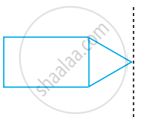Exercise 1.1 | Q 2 | Page 4

Draw the mirror image:Exercise 1.1 | Q 3 | Page 4

Draw the mirror image: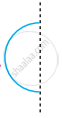Exercise 1.1 | Q 4 | Page 4

Draw the mirror image:Exercise 1.1 | Q 5 | Page 4

Draw the mirror image: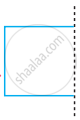Exercise 1.2 [Page 5]

### Tamil Nadu Board Samacheer Kalvi solutions for Class 4th Mathematics Term 2 Answers Guide Chapter 1 GeometryExercise 1.2 [Page 5]

Exercise 1.2 | Q A. 1. | Page 5

Draw the line of symmetry in the following figure.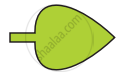Exercise 1.2 | Q A. 2. | Page 5

Draw the line of symmetry in the following figure.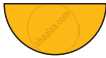Exercise 1.2 | Q A. 3. | Page 5

Draw the lines of symmetry in the following figure.Exercise 1.2 | Q A. 4. | Page 5

Draw the line of symmetry in the following figure.Exercise 1.2 | Q B. 1. | Page 5

How many lines of symmetry for the following figureExercise 1.2 | Q B. 2. | Page 5

How many lines of symmetry for the following figure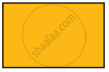Exercise 1.2 | Q B. 3. | Page 5

How many lines of symmetry for the following figureExercise 1.2 | Q B. 4. | Page 5

How many lines of symmetry for the following figureExercise 1.3 [Page 6]

### Tamil Nadu Board Samacheer Kalvi solutions for Class 4th Mathematics Term 2 Answers Guide Chapter 1 GeometryExercise 1.3 [Page 6]

Exercise 1.3 | Q A. 1. | Page 6

Identify the following figure is symmetrical about dotted line?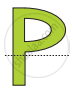Exercise 1.3 | Q A. 2. | Page 6

Identify the following figure is symmetrical about dotted line?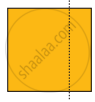Exercise 1.3 | Q A. 3. | Page 6

Identify the following figure is symmetrical about dotted line?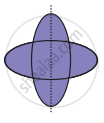Exercise 1.3 | Q A. 4. | Page 6

Identify the following figure is symmetrical about dotted line?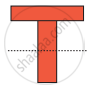Exercise 1.3 | Q A. 5. | Page 6

Identify the following figure is symmetrical about dotted line?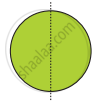Exercise 1.3 | Q 2.1 | Page 6

Who am I?

I have no side, no vertices and many lines of symmetry. ______

Exercise 1.3 | Q 2.2 | Page 6

Who am I?

I have 4 equal sides and 4 lines of symmetry. ______

Exercise 1.3 | Q 2.3 | Page 6

Who am I?

I have 3 equal sides, 3 vertices and 3 lines of symmetry ______

Exercise 1.3 | Q 2.4 | Page 6

Who am I?

I have 4 sides opposite sides are equal and I have 2 lines of symmetry ______

## Chapter 1: Geometry

Exercise 1.1Exercise 1.2Exercise 1.3## Tamil Nadu Board Samacheer Kalvi solutions for Class 4th Mathematics Term 2 Answers Guide chapter 1 - Geometry

Tamil Nadu Board Samacheer Kalvi solutions for Class 4th Mathematics Term 2 Answers Guide chapter 1 (Geometry) include all questions with solution and detail explanation. This will clear students doubts about any question and improve application skills while preparing for board exams. The detailed, step-by-step solutions will help you understand the concepts better and clear your confusions, if any. Shaalaa.com has the Tamil Nadu Board of Secondary Education Class 4th Mathematics Term 2 Answers Guide solutions in a manner that help students grasp basic concepts better and faster.

Further, we at Shaalaa.com provide such solutions so that students can prepare for written exams. Tamil Nadu Board Samacheer Kalvi textbook solutions can be a core help for self-study and acts as a perfect self-help guidance for students.

Concepts covered in Class 4th Mathematics Term 2 Answers Guide chapter 1 Geometry are Concept of Symmetry, Lines of Symmetry for Regular Polygons, Concept of Reflection Symmetry, Viewing Different Sections of a Solid.

Using Tamil Nadu Board Samacheer Kalvi Class 4th solutions Geometry exercise by students are an easy way to prepare for the exams, as they involve solutions arranged chapter-wise also page wise. The questions involved in Tamil Nadu Board Samacheer Kalvi Solutions are important questions that can be asked in the final exam. Maximum students of Tamil Nadu Board of Secondary Education Class 4th prefer Tamil Nadu Board Samacheer Kalvi Textbook Solutions to score more in exam.

Get the free view of chapter 1 Geometry Class 4th extra questions for Class 4th Mathematics Term 2 Answers Guide and can use Shaalaa.com to keep it handy for your exam preparation